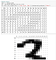# fast.ai 2020 — Lesson 3

fastai/fastbook

`# Load saved learnerlearn_inf = load_learner(path/'export.pkl')# Predict given imagelearn_inf.predict('images/grizzly.jpg')# See labelslearn_inf.dls.vocab`

Jupyter Notebook widgets are created using IPython widgets.

Deploying CPU is easier than GPU in most of the cases. GPU is needed only if the deployed model still requires a lot of computation like some video model or if the model gets thousands of requests every second making it possible to batch them.

Out of domain data = data might be too optimistic. For example when predicting bears the images might be too clear and middle of the image when in the real use case the bear might be small and it might be night.

Domain shift = a model is trained using certain kind of data that is not anymore relevant because of some change.

It’s impossible to know exactly what a machine learning model is going to do the same way it’s simple to understand what a program will do by looking the source code. To avoid problems in deploying ML model it’s good to use following checklist.

• Try model yourself to see that it makes sense in high level
• Deploy limited time or geography
• Gradually expand the number of users/usecases and have good reporting in place

fastai/fastbook

Predict MNIST data but only images with label 3 or 7. Black and white images with 28x28 pixels.

People who have used Python tend to use Numpy array instead of tensor but it’s recommended to start to use tensor because it’s much faster in many cases.This is what the dataset looks like when also printing pixel color value

Baseline is a simple model that is better than random. For this task Jeremy’s baseline was to calculate an average to each pixel.

Sometimes people show how amazing their models are but then always predicting mean beats the model.

`/255` is because the values should be between 0 and 1.

Broadcasting means that tensor is copied over certain dimension making it the same dimension as some other tensor. For example if one number is multiplied by an array behind the scenes the number changes to similar size array with that number in every position.

The above thing is the same as:

`tensor([1,2,3]) + 1`

Many things could be done using loops but using matrices is much faster.

Really simple baseline gives already 95% accuracy

This is not machine learning because the definition we looked previously said that we modify some parameters in every loop. The next there is something still very simple but machine learning.

`def pr_eight(x,w) = (x*w).sum()`

This code uses weight (w) to multiple the pixels and then it sums up the values. This way weights can be used in different places to increase or decrease the number but pixels affect the outcome.

1. Initialize the weights
2. Predict is an image three or seven
3. Calculate how much off the predictions were
4. Calculate gradient (how changing the weight affect loss)
5. Update weights based on the gradient
6. Back to predict (repeat this loop multiple times)
`# Every time xt do calculation it should remember what it was# and then later it's easy to take derivative of that.xt = tensor(3.).requires_grad_()# Just some functiondef f(x): return x**2# Give xt to the functionyt = f(xt)yt# Prints => tensor(9., grad_fn=<PowBackward0>)# backward takes the derivative of the previous calculationyt.backward()# And just to print the derivativext.grad()# Prints => tensor(6.)`

The same thing but with rank two tensor

`xt = tensor([3.,4.,10.]).requires_grad_()def f(x): return (x**2).sum()yt = f(xt)yt.backward()xt.grad# Prints => tensor([ 6.,  8., 20.])`

Update weights using gradient: `w -= gradient(w) * lr`

lr = learning rate = some small number like 0.01

Learning rate affects step size.

PyTorch has `.data` function for tensors which can be used when we don't want to calculate the gradient. This is used when we update weights.

Gradient need to be cleared after each step `params.grad = None`

Accuracy doesn’t work as a loss function because if we change some weight only a little bit it doesn’t necessary affect any prediction.